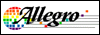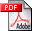1 A1301 Continuous-Time Ratiometric Linear Hall Effect Sensors 300K2 A1301 Continuous-Time Ratiometric Linear Hall Effect Sensors 273K3 A1301_06 Continuous-Time Ratiometric Linear Hall Effect Sensors 273K4 A1301ELHLT Continuous-Time Ratiometric Linear Hall Effect Sensors 300K5 A1301ELHLT-T Continuous-Time Ratiometric Linear Hall Effect Sensors 300K6 A1301ELHLT-T Continuous-Time Ratiometric Linear Hall Effect Sensors 273K7 A1301EUA Continuous-Time Ratiometric Linear Hall Effect Sensors 300K8 A1301EUA-T Continuous-Time Ratiometric Linear Hall Effect Sensors 300K9 A1301EUA-T Continuous-Time Ratiometric Linear Hall Effect Sensors 273K10 A1301KLHLT Continuous-Time Ratiometric Linear Hall Effect Sensors 300K11 A1301KLHLT-T Continuous-Time Ratiometric Linear Hall Effect Sensors 300K12 A1301KLHLT-T Continuous-Time Ratiometric Linear Hall Effect Sensors 273K13 A1301KUA Continuous-Time Ratiometric Linear Hall Effect Sensors 300K14 A1301KUA-T Continuous-Time Ratiometric Linear Hall Effect Sensors 300K15 A1301KUA-T Continuous-Time Ratiometric Linear Hall Effect Sensors 273K